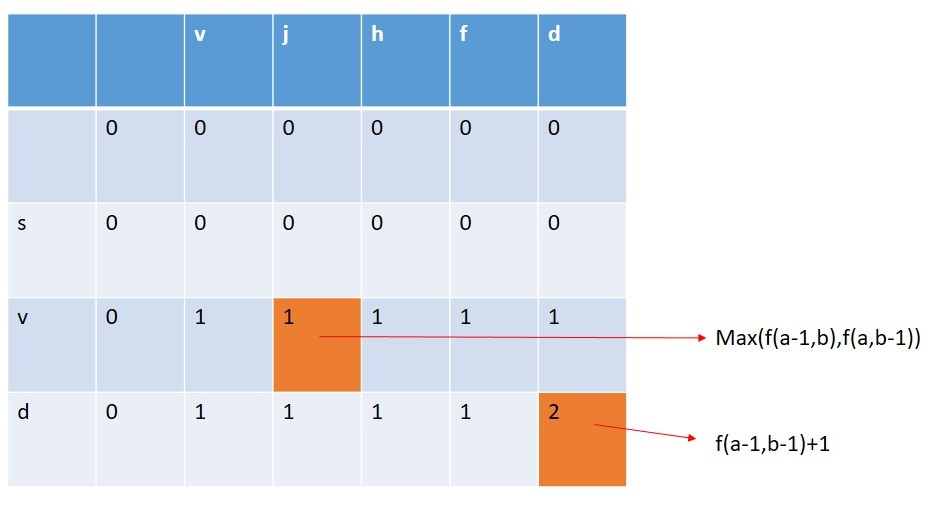Q:

# Printing Longest Common Subsequence

Given two strings, you have to find and print the longest common subsequence between them.

```    Input:
T Test case
T no of input string will be given to you.

E.g.
3

abcd abxy
sghk rfgh
svd vjhfd

Constrain
1≤ length (string1) ≤100
1≤ length (string2) ≤100

Output:
Print the length of the longest common subsequence formed from these two strings.
```

Example

```    T=3

Input:
abcd abxy

Output:
2 (xy)

Input:
sghk rfgh

Output:
2 (gh)

Input:
svd vjhfd

Output:
2 (vd)```

Let there are two strings str1 and str2.

```    str1 = "abcd"
str2 = "abxy"
```

Using a dynamic programming algorithm to find the longest common subsequence between two given string is very efficient and fast as compared to the recursion approach.

Let f(a,b) = count the number of common subsequence from the two string starting from 0 to position a and starting from 0 to position b.

Considering the two facts:

1. If the character of string1 at index a and character of string1 at index b are the same then we have to count how many characters are the same between the two strings before these indexes? Therefore,
```    f(a,b)=f(a-1,b-1)+1
```
2. If the character of string1 at index a and character of string1 at index b are not same then we have to calculate the maximum common character count between (0 to a-1 of string1 and 0 to b of string2) and (0 to a of string1 and 0 to b-1 of string2).
```    f(a,b) = max(f(a-1,b),f(a,b-1))
```

For the two strings:

```    str1 = "abcd"
str2 = "abxy"
```C++ Implementation:

``````#include <bits/stdc++.h>
using namespace std;

int count(string str1, string str2)
{
int len1 = str1.length();
int len2 = str2.length();
int arr[len1 + 1][len2 + 1];
memset(arr, 0, sizeof(arr));
for (int i = 1; i <= len1; i++) {
for (int j = 1; j <= len2; j++) {
if (str1[i - 1] == str2[j - 1]) {
arr[i][j] = arr[i - 1][j - 1] + 1;
}
else {
arr[i][j] = max(arr[i - 1][j], arr[i][j - 1]);
}
}
}
return (arr[len1][len2]);
}

int main()
{
int t;
cout << "Test Case: ";
cin >> t;
while (t--) {
string str1, str2;
cout << "Enter the two strings: ";
cin >> str1 >> str2;
cout << "length of the Shorest SubString: " << count(str1, str2) << endl;
}
return 0;
}``````

Output

```Test Case: 3
Enter the two strings: abcd abxy
length of the Shorest SubString: 2
Enter the two strings: sghk rfgh
length of the Shorest SubString: 2
Enter the two strings: svd svjhfd
length of the Shorest SubString: 3```